# find all solutions in the interval [0,2 pi) sin(x+(3.14/3) + sin(x- 3.14/3) =1 sin^4 x cos^2 x Since sin (a+b) = sina cosb + cosb sina and sin (a-b) = sina cosb - cosb sina, the first problem can be written 2 sin x cos (pi/3)= sin x The soluti related homework questions

• #### find all solutions in the interval [0,2 pi) sin(x+(3.14/3) + sin(x- 3.14/3) =1 sin^4 x cos^2 x Since sin (a+b) = sina cosb + cosb sina and sin (a-b) = sina cosb - cosb sina, the first problem can be written 2 sin x cos (pi/3)= sin x The soluti

find all solutions in the interval [0,2 pi) sin(x+(3.14/3) + sin(x- 3.14/3) =1 sin^4 x cos^2 xSince sin (a+b) = sina cosb + cosb sina and sin (a-b) = sina cosb - cosb sina, the first problem can be written 2 sin x cos (pi/3)= sin x The solution to sin x = 1 is x = pi/2 For your other problem sin^4 cos^2 x = sin^4 x(1 - sin^2x)=0 The solutions are sin x = 0 a...

• #### It’s review question, I need this as soon as possible. Thank you 3) For thè diferential equation: (a) The point zo =-1 is an ordinary point. Compute the recursion formula for the coefficients of...It’s review question, I need this as soon as possible. Thank you 3) For thè diferential equation: (a) The point zo =-1 is an ordinary point. Compute the recursion formula for the coefficients of the power series solution centered at zo- -1 and use it to compute the first three nonzero terms of the power series when -1)-s and v(-1)-0. (25 points) (b) Th...

• #### In any angle ABC prove tht the perimeter = a/Sin A(Sin A + Sin B + Sin C) Start with perimeter = a+b+c but b= aSinB/sinA and c= aSinC/SinA and if you dont remember, a=a=a*SinA/ SinA

In any angle ABC prove tht the perimeter = a/Sin A(Sin A + Sin B + Sin C)Start with perimeter = a+b+c but b= aSinB/sinA and c= aSinC/SinA and if you dont remember, a=a=a*SinA/ SinA

• #### Dont copié formé thé book oh ya dont copié formé thé book cause you Oiil inde up being triste soi remembré not toi copié frome thé book oh ya

Dont copié formé thé book oh ya dont copié formé thé book cause you Oiil inde up being triste soi remembré not toi copié frome thé book oh ya!translation in english please!

• #### How would you establish this identity: (1+sec(beta))/(sec(beta))=(sin^2(beta))/(1-cos(beta)) on the right, sin^2 = 1-cos^2, that factor to 1-cos * `1+cos, then the denominator makes the entire right side 1+cosB which is 1+1/sec which is 1/sec (sec+1)

How would you establish this identity: (1+sec(beta))/(sec(beta))=(sin^2(beta))/(1-cos(beta))on the right, sin^2 = 1-cos^2, that factor to 1-cos * `1+cos, then the denominator makes the entire right side 1+cosB which is 1+1/sec which is 1/sec (sec+1) qedusing sec(beta) = 1/cos(beta): 1+sec(beta))/(sec(beta))= 1 + cos(beta) sin^2(beta)/(1-cos(beta)) = (1-cos^2...

• #### 2. A dragon is flying around in a pattern given by the parametric curve r(t) (cos(t) cos((sin(t) sin(t) cos(t)j. cos(t) - cos sin(t)-sin(t) cos(t))j (a) Find a formula for the velocity of the dragon...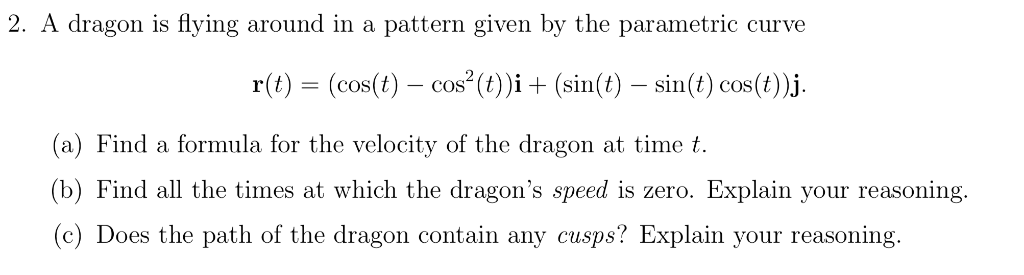2. A dragon is flying around in a pattern given by the parametric curve r(t) (cos(t) cos((sin(t) sin(t) cos(t)j. cos(t) - cos sin(t)-sin(t) cos(t))j (a) Find a formula for the velocity of the dragon at time t (b) Find all the times at which the dragon's speed is zero. Explain your reasoning. c) Does the path of the dragon contain any cusps? Explain your...

• #### Solve the following equation for 0 less than and/or equal to "x" less than and/or equal to 360 -- cos^2x - 1 = sin^2x -- Attempt: cos^2x - 1 - sin^2x = 0 cos^2x - 1 - (1 - cos^2x) = 0 cos^2x - 1 - 1 + cos^2x = 0 2cos^2x - 2 = 0 (2cos^2x/2)= (-2/2) cos^

Solve the following equation for 0 less than and/or equal to "x" less than and/or equal to 360--cos^2x - 1 = sin^2x--Attempt:cos^2x - 1 - sin^2x = 0cos^2x - 1 - (1 - cos^2x) = 0cos^2x - 1 - 1 + cos^2x = 02cos^2x - 2 = 0(2cos^2x/2)= (-2/2)cos^2x = -1cosx = square root of -1And I can't do anything with this now...what am I doing wrong?--Textbook answers:0, 180...

• #### The only ideas that can be used include: area ABCI-RA2(A+B+C-lpi), the Pythagorean theorem: Cos c cos a cos b. Vectors-dot product cross product, sin A-sin a/sin c; cos A-cos a sin b/sin c; spher...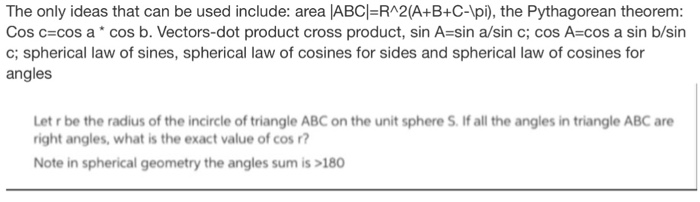The only ideas that can be used include: area ABCI-RA2(A+B+C-lpi), the Pythagorean theorem: Cos c cos a cos b. Vectors-dot product cross product, sin A-sin a/sin c; cos A-cos a sin b/sin c; spherical law of sines, spherical law of cosines for sides and spherical law of cosines for angles Let r be the radius of the incircle of triangle ABC on the unit sp...

• #### the original problem was: (sin x + cos x)^2 + (sin x - cos x)^2 = 2 steps too please I got 1 for (sin x + cos x)^2 but then what does (sin x - cos x)^2 become since it's minus

the original problem was: (sin x + cos x)^2 + (sin x - cos x)^2 = 2steps too pleaseI got 1 for (sin x + cos x)^2 but then what does (sin x - cos x)^2 become since it's minus?

• #### 1) 1+cos(3t)/ sin(3t) + sin(3t)/( 1+ cos(3t))= 2csc(3t) 2) sec^2 2u-1/ sec^2 2u= sin^2 2u 3) cosB/1- sinB= secB+ tanB

1) 1+cos(3t)/ sin(3t) + sin(3t)/( 1+ cos(3t))= 2csc(3t)2) sec^2 2u-1/ sec^2 2u= sin^2 2u3) cosB/1- sinB= secB+ tanB

• #### convert to radians 5) γ 4cos (2θ-5) r 3 (sin(e - 2))2 + 4 cos(20-5) r-6(sin(9-2))2 + 4 cos(2e-5) 9(sin(9-2))2 + 4 cos(2e-5) r 12(sin(e - 2))2 +4 cos(20 5) 5) γ 4cos (2θ-5) r 3 (sin(e - 2))2 +...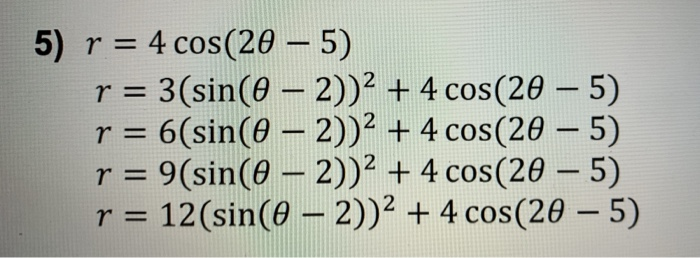convert to radians 5) γ 4cos (2θ-5) r 3 (sin(e - 2))2 + 4 cos(20-5) r-6(sin(9-2))2 + 4 cos(2e-5) 9(sin(9-2))2 + 4 cos(2e-5) r 12(sin(e - 2))2 +4 cos(20 5) 5) γ 4cos (2θ-5) r 3 (sin(e - 2))2 + 4 cos(20-5) r-6(sin(9-2))2 + 4 cos(2e-5) 9(sin(9-2))2 + 4 cos(2e-5) r 12(sin(e - 2))2 +4 cos(20 5)

• #### prove that sinA/(1+cosA) + (1+cosA)/(sinA) = 2cosecA

prove that sinA/(1+cosA) + (1+cosA)/(sinA) = 2cosecA

• #### 2. Study convergence of the integral + log r 00-1-sinr π (π-x) log z o log(1-sina) 2. Study convergence of the integral + log r 00-1-sinr π (π-x) log z o log(1-sina)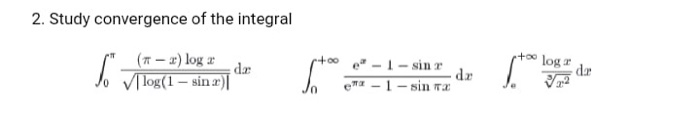2. Study convergence of the integral + log r 00-1-sinr π (π-x) log z o log(1-sina) 2. Study convergence of the integral + log r 00-1-sinr π (π-x) log z o log(1-sina)

• #### The identity of: sin^4x+cos^4x= (sin^2x+cos^2x)(sin^2x+cos^2x) sin^2x+cos^2=1 This is the answer I come up with, it is not one of the options available as an answer

The identity of: sin^4x+cos^4x= (sin^2x+cos^2x)(sin^2x+cos^2x) sin^2x+cos^2=1 This is the answer I come up with, it is not one of the options available as an answer. The answers given are 1. -2sin^2xcos^2x 2. 1+2sin^2x-2sin^4x 3. 1+3sin^3x-2sin^2x 4. 1-2sin^2x+2sin^4x 5. 0

• #### cos(tan + cot) = csc only simplify one side to equal csc so far I got this far: [((cos)(sin))/(cos)] + [((cos)(cos))/(sin)] = csc I don't know what to do next

cos(tan + cot) = csconly simplify one side to equal cscso far I got this far: [((cos)(sin))/(cos)] + [((cos)(cos))/(sin)] = cscI don't know what to do next

• #### 2) Use the sum and difference identites sin[x + pi/4] + sin[x-pi/4] = -1 sinx cospi/4 + cosxsin pi/4 + sinx cos pi/4 - cosx sin pi/4 = -1 2 sin x cos pi/4 =-1 cos pi/4 = sqr2/2 2sin^x(sqrt2/2) = -1 sin x = -sqrt2 x = 7pi/4 and 5pi/4 Am I co

2) Use the sum and difference identites sin[x + pi/4] + sin[x-pi/4] = -1 sinx cospi/4 + cosxsin pi/4 + sinx cos pi/4 - cosx sin pi/4 = -1 2 sin x cos pi/4 =-1 cos pi/4 = sqr2/2 2sin^x(sqrt2/2) = -1 sin x = -sqrt2 x = 7pi/4 and 5pi/4 Am I correct?

• #### if sinx=(4/5), where 0 degrees<x<90 degrees, find the value of cos (x+180) 25+25+100+n=250 since x is in the first quadrant, then x+180º is in the third quadrant, and cos(x+180) = =cos x You should recognize the 3,4,5 right-angled triangle and c

if sinx=(4/5), where 0 degrees<x<90 degrees, find the value of cos (x+180)25+25+100+n=250since x is in the first quadrant, then x+180º is in the third quadrant, and cos(x+180) = =cos x You should recognize the 3,4,5 right-angled triangle and cosx = 3/5 so cos(x+180)=-3/53x+y=90+2x+y=90

• #### Let g(x) = sin (cos x^3) Find g ' (x): The choices are a) -3x^2sinx^3cos(cos x^3) b) -3x^2sinx^3sin(cos x^3) c) -3x^2cosx^3sin(cos x^3) d) 3x^2sin^2(cos x^3) I'm not exactly sure where I should start

Let g(x) = sin (cos x^3) Find g ' (x):The choices are a) -3x^2sinx^3cos(cos x^3)b) -3x^2sinx^3sin(cos x^3)c) -3x^2cosx^3sin(cos x^3)d) 3x^2sin^2(cos x^3)I'm not exactly sure where I should start.Should I begin with d/dx of sin? Or do the inside derivative first...and do I have to separate cos and x^3 as well?

• #### sin^2 theta + 2 cos theta -1/ (sin^2 theta +3 cos theta-3= cos^2 theta+cos theta/ (-sin^2 theta) Prove the identity

sin^2 theta + 2 cos theta -1/ (sin^2 theta +3 cos theta-3= cos^2 theta+cos theta/ (-sin^2 theta)Prove the identity.

• #### ANSWER 3: Deduction:-14.285714285714390 -300e 800t cos (600t) 384e-800f sin(600t) mA cos (600t) 192e-800f sin(600t) mA -300e 800t cos (600t) - 192e-800f sin(600t) mA -600e 800t cos (600t) 192e-800f s...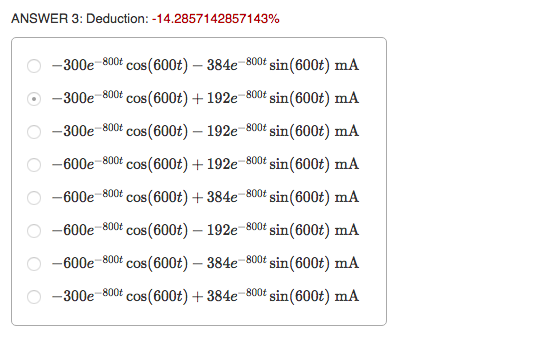ANSWER 3: Deduction:-14.285714285714390 -300e 800t cos (600t) 384e-800f sin(600t) mA cos (600t) 192e-800f sin(600t) mA -300e 800t cos (600t) - 192e-800f sin(600t) mA -600e 800t cos (600t) 192e-800f sin(600t) mA -600e 800t cos(600t) 384e-800f sin(600t) mA -600e 800t cos (600t) - 192e-800f sin(600t) mA -600e 800t cos (600t) 384e-800f sin(600t) mA -300e 800t c...